Get inspired by the success stories of our students in IIT JAM MS, ISI  MStat, CMI MSc DS.  Learn More

# ISI MStat 2016 Problem 1 | Area bounded by the curves | PSB SampleThis is a beautiful problem from ISI MStat 2016 Problem 1, PSB Sample, based on area bounded by the curves. We provide a detailed solution with the prerequisites mentioned explicitly.

## Problem- ISI MStat 2016 Problem 1

In the diagram below,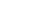is a straight line that intersects the graph of a polynomial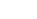of degree 2 at the points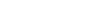and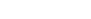The area of the shaded region is 36 square units. Obtain the expression for.

## Prerequisites

• Area bounded by the curve
• Polynomials of degree 2
• Area of a triangle

## Solution

Let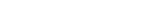as givenis of degree 2 .

Now from the figure we can see thatintersectat pointsandHence we have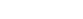and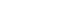, which gives ,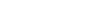---(1) and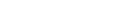----(2)

Then ,

See from Fig-1 we can say that Area of the shaded region = (Area bounded by the curve P(x) and x-axis )- (Area of the triangle ABC) - (Area bounded by the curve P(x) , x=5 and x=L )

=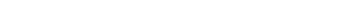=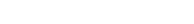-36

=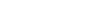-36

Again it is given that area of the shaded region is 36 square units.

So,-36 =36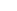=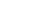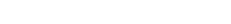. After integration we get ,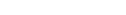---(3)

Now we have three equations and three unknowsSolving this three equations by elimination and substitution we get ,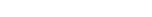Therefore , the expression foris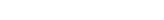.

## Previous MStat Posts:

This is a beautiful problem from ISI MStat 2016 Problem 1, PSB Sample, based on area bounded by the curves. We provide a detailed solution with the prerequisites mentioned explicitly.

## Problem- ISI MStat 2016 Problem 1

In the diagram below,is a straight line that intersects the graph of a polynomialof degree 2 at the pointsandThe area of the shaded region is 36 square units. Obtain the expression for.

## Prerequisites

• Area bounded by the curve
• Polynomials of degree 2
• Area of a triangle

## Solution

Letas givenis of degree 2 .

Now from the figure we can see thatintersectat pointsandHence we haveand, which gives ,---(1) and----(2)

Then ,

See from Fig-1 we can say that Area of the shaded region = (Area bounded by the curve P(x) and x-axis )- (Area of the triangle ABC) - (Area bounded by the curve P(x) , x=5 and x=L )

==-36

=-36

Again it is given that area of the shaded region is 36 square units.

So,-36 =36=. After integration we get ,---(3)

Now we have three equations and three unknowsSolving this three equations by elimination and substitution we get ,Therefore , the expression foris.

## Previous MStat Posts:

This site uses Akismet to reduce spam. Learn how your comment data is processed.

### Knowledge Partner